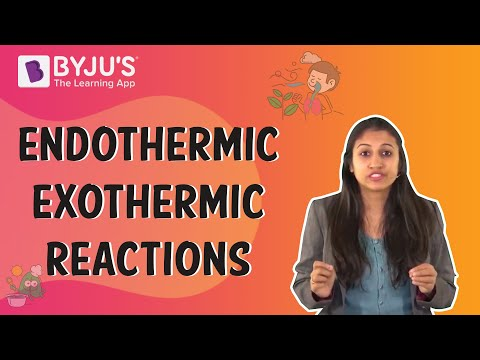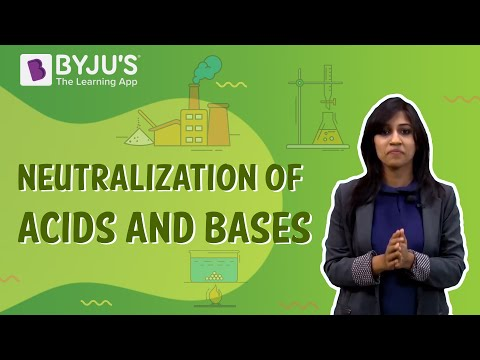Jet Set Go! All about Aeroplanes Jet Set Go! All about Aeroplanes

# Exothermic Reactions Questions

The reactions in which the energy is released as a result of the reaction are called Exothermic reactions. The products formed in the exothermic reactions have stronger bonds than those present in the reactants. The value of the overall standard enthalpy change (ΔHo) is negative for the exothermic reactions.

 Definition: The reactions which give off energy in the form of heat after completion are called Exothermic reactions.

## Exothermic Reactions Chemistry Questions with Solutions

Q1: Which of the following reactions is an exothermic reaction?

(i) CaO (s) + H2O (l) → Ca(OH)2 (aq) + Δ

(ii)

$$\begin{array}{l}2AgCl (s) \overset{\Delta }{\rightarrow}2Ag (s) + Cl_{2}(g)\end{array}$$
1. (i)
2. (ii)
3. Both (i) and (ii)
4. None of the above

Explanation: Since heat (Δ) is shown at the side of the products, this indicates that heat is produced as a result of the reaction. Hence, as the heat is released, the reaction is an exothermic reaction.

Q2. Comment whether the reaction 2Pb(NO3)2 (s) → 2PbO (s) + 4NO (g) + O2 (g) is exothermic or not and give reason for your answer.

Answer: In this reaction, 2Pb(NO3)2 undergoes decomposition to form 3 products. It is to be noted that decomposition reactions always proceed by gaining some amount of energy. This is because the breaking of bonds requires some amount of energy. Hence, any example of a decomposition reaction is an exothermic reaction too.

Q3. Which of the following is the most suitable to carry out a reaction in which the temperature has to be monitored?

1. A polystyrene cup
2. A glass beaker
3. A burette
4. A measuring cylinder

Explanation: This is because the polystyrene cup helps to insulate the reaction mixture from temperature or heat changes. Hence, a reaction carried out in a polystyrene cup (covered) will be unaffected from the heat changes in the surroundings.

Q4. Determine which one of these is an endothermic reaction.

1. Oxidation of Mg
2. Combustion
3. Thermal Decomposition
4. All of the above

Explanation: Thermal Decomposition is the decomposition in the presence of heat.

Q5. Is the burning of coke in air an example of exothermic reaction or endothermic reaction?

Answer: The burning of coke in air is a combustion reaction.

C (coke) + O2 → CO2

The burning of coke is accompanied by the evolution of heat and light. Thus, since the energy in the form of heat and light is given out, the reaction is exothermic.

Q6. Why does the reaction vessel used in the reaction between ammonium chloride and barium hydroxide become cold? What kind of a reaction is this?

Answer: The vessel used in the reaction between ammonium chloride and barium hydroxide becomes cold because the reactants absorb the energy required for their reaction to occur from their surroundings. Since, the reactants are taken in the reaction vessel, they absorb the energy from the reaction vessel and hence the vessel feels cold. This is an endothermic reaction as the heat is absorbed in the reaction.

Q7. What is a State function?

Answer: A state function is defined as any physical quantity that depends merely on the state of the system and not on the path taken by which that state has been attained. For example: potential energy is a state function since the value of potential energy is independent of the work done.

Q8. What does the negative change in energy on an energy level diagram indicate?

Answer: The negative energy change indicates that as the reaction proceeds, the energy of the reaction mixture decreases. This implies that the energy of the new products formed is lesser than the energy of the reactants. Hence, ΔH is negative for the given reaction and the reaction is exothermic.

Q9. In the energy profile diagram, what does the height of the maxima indicate?

Answer: The energy vs reaction coordinate curve indicates the amount of energy of reactants and the products along with the energy of intermediates or transition states (if any). Every reaction requires a certain minimum amount of energy to proceed further. This energy is called the activation energy. Since the activation energy has the highest value for any reaction in the energy profile diagram, the height of the maxima indicates the activation energy.

Q10. What can you conclude from the energy profile diagram below?

Answer: The energy of the products is higher than the energy of the reactants. This implies that energy has been absorbed through the reaction. The reaction must be endothermic.

Q11. If the energy released when the products are formed is more than the amount of energy absorbed by the reactants, comment on the strength of bonds of reactants and products.

Answer: During a chemical reaction, if the energy absorbed is lesser than the energy released, the bonds of reactants are weaker than those of products’ bonds. This is because the amount of energy released or absorbed is associated with the strength of bonds. The stronger the bond the higher is the energy required to break it. Hence, for products, the stronger the bond, the more stable the compound will be. The stability of a compound is associated with its energy. Hence, greater amount of energy released accounts for higher strength of the bonds of the products.

Q12. What is meant by a thermite reaction? Give an example.

Answer: The thermite reactions involve the reduction of metal oxides by the use of highly reactive metals such as sodium (Na) and aluminium (Al). These reactions are highly exothermic. An example of these reactions is the reaction between iron oxide and aluminium. In this reaction, aluminium displaces iron from its oxide. This reaction produces so much heat that the product of reaction i.e. iron is obtained in its molten form.

Al (s) + Fe2O3 (s) → Al2O3 (s) + Fe (l) + Δ

Q13. What is the difference between a liquid and an aqueous solution?

Answer: A liquid is one of the three states of matter which has intermediate properties between those of solids and gases. While an aqueous solution involves a solute dissolved in a liquid taken as solvent.

Q14. Is respiration an endothermic reaction or an exothermic reaction?

Answer: Respiration is a kind of combustion reaction. During respiration, the sugar molecules break up within the cells in the presence of oxygen to form heat and energy that is utilised by our body for carrying out several tasks. Hence, respiration is an exothermic reaction.

Q15. The half-life of a reaction decreases when the temperature of the reaction changes from T1 to T2. Pick a correct option for this reaction about the temperature and the type of reaction.

1. T1<T2 and the reaction is endothermic
2. T1<T2 and the reaction is exothermic
3. T1>T2 and the reaction is endothermic
4. T1>T2 and the reaction is exothermic

Explanation: The exothermic reactions are favoured at a low temperature. When the temperature of the system decreases, the equilibrium shifts itself in order to counteract the decrease in the temperature. Hence, more heat is released at the end of the reaction and the half-life of the reaction is decreased. Thus, T1>T2 and the reaction is exothermic.

## Practise Questions on Exothermic Reactions

Q1. Why is the neutralization reaction an exothermic reaction?

Q2. Give an example of a reaction that is both a combination reaction and an exothermic reaction. Give an equation for the reaction.

Q3. How can we determine that the reaction is either endothermic or exothermic?

Q4. What is the correct way to dilute an acid?

Q5. During the production of slaked lime, heat is evolved from the reaction. Are all reactions that involve the formation of a base exothermic?

Click the PDF to check the answers for Practice Questions.

## Endothermic and Exothermic Reactions## Neutralization of Acids and Bases